# 5 2 Properties of Quadratic Functions in Standard

• Slides: 19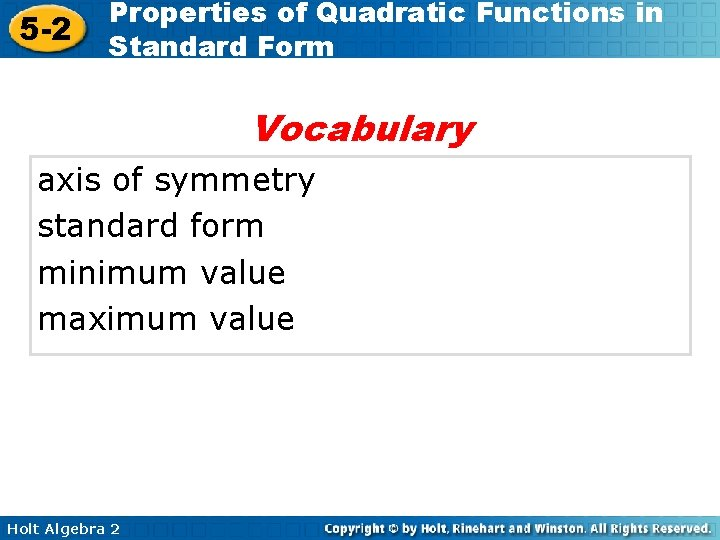5 -2 Properties of Quadratic Functions in Standard Form Vocabulary axis of symmetry standard form minimum value maximum value Holt Algebra 2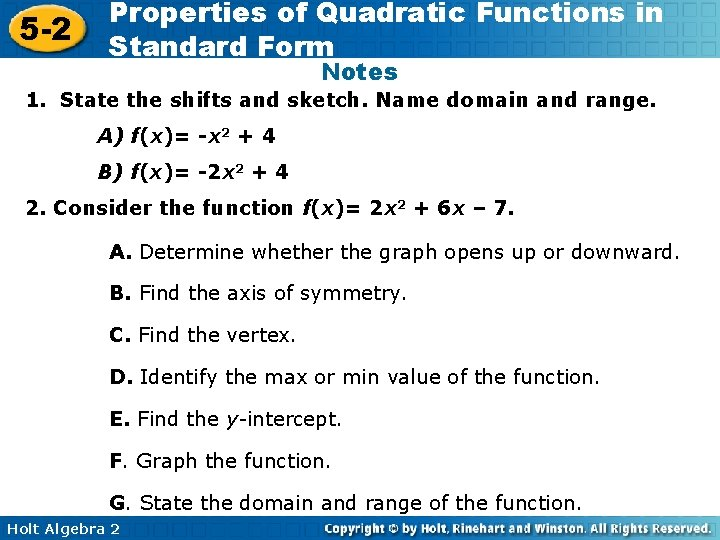5 -2 Properties of Quadratic Functions in Standard Form Notes 1. State the shifts and sketch. Name domain and range. A) f(x)= -x 2 + 4 B) f(x)= -2 x 2 + 4 2. Consider the function f(x)= 2 x 2 + 6 x – 7. A. Determine whether the graph opens up or downward. B. Find the axis of symmetry. C. Find the vertex. D. Identify the max or min value of the function. E. Find the y-intercept. F. Graph the function. G. State the domain and range of the function. Holt Algebra 25 -2 Properties of Quadratic Functions in Standard Form This shows that parabolas are symmetric curves. The axis of symmetry is the line through the vertex of a parabola that divides the parabola into two congruent halves. Holt Algebra 2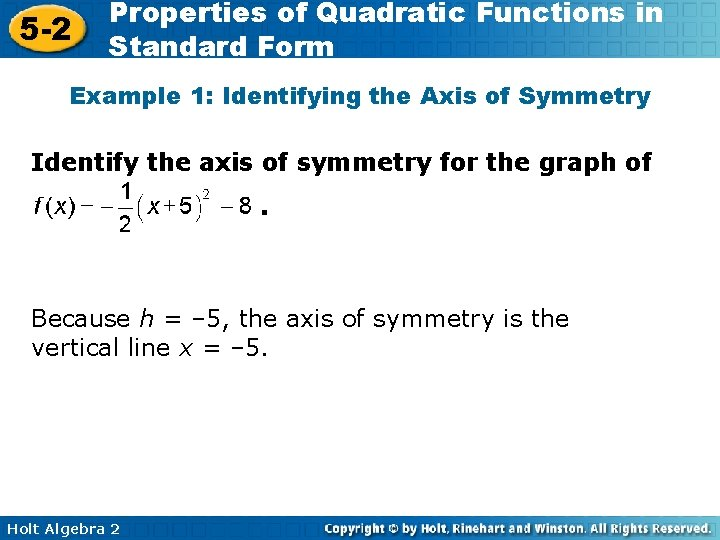5 -2 Properties of Quadratic Functions in Standard Form Example 1: Identifying the Axis of Symmetry Identify the axis of symmetry for the graph of. Because h = – 5, the axis of symmetry is the vertical line x = – 5. Holt Algebra 2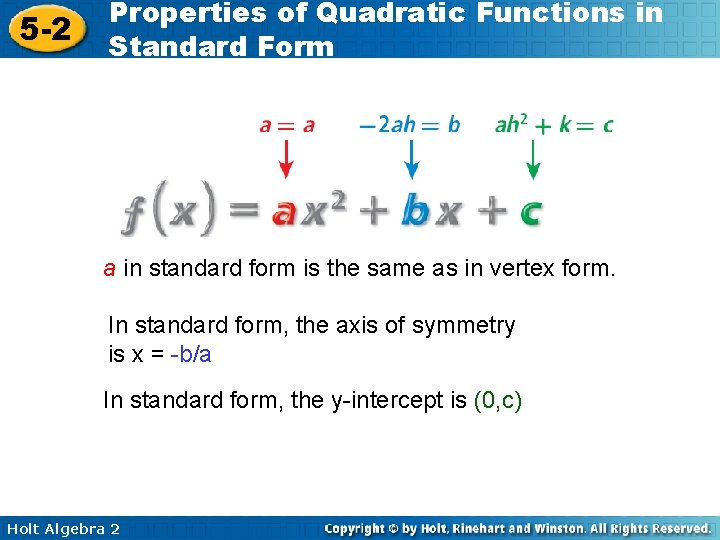5 -2 Properties of Quadratic Functions in Standard Form a in standard form is the same as in vertex form. In standard form, the axis of symmetry is x = -b/a In standard form, the y-intercept is (0, c) Holt Algebra 2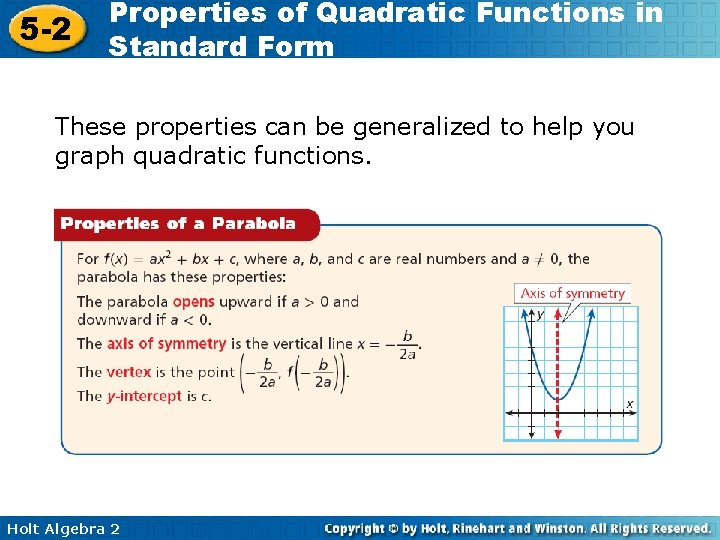5 -2 Properties of Quadratic Functions in Standard Form These properties can be generalized to help you graph quadratic functions. Holt Algebra 2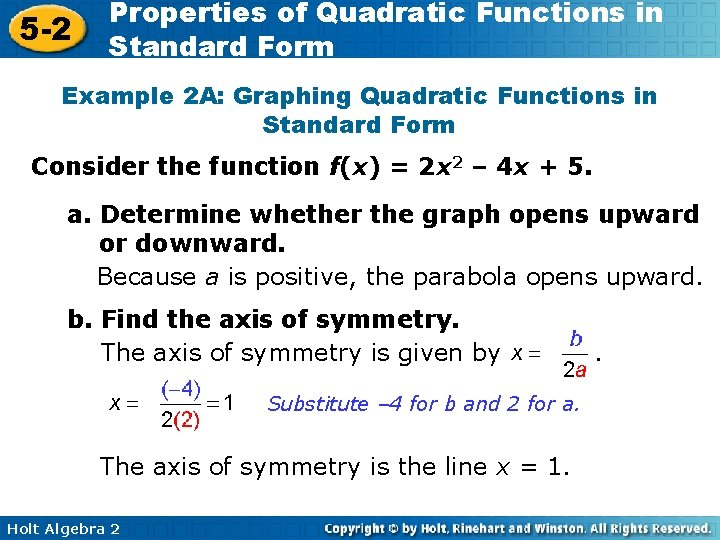5 -2 Properties of Quadratic Functions in Standard Form Example 2 A: Graphing Quadratic Functions in Standard Form Consider the function f(x) = 2 x 2 – 4 x + 5. a. Determine whether the graph opens upward or downward. Because a is positive, the parabola opens upward. b. Find the axis of symmetry. The axis of symmetry is given by Substitute – 4 for b and 2 for a. The axis of symmetry is the line x = 1. Holt Algebra 2 .5 -2 Properties of Quadratic Functions in Standard Form Example 2 A: Graphing Quadratic Functions in Standard Form Consider the function f(x) = 2 x 2 – 4 x + 5. c. Find the vertex. The vertex lies on the axis of symmetry, so the x-coordinate is 1. The y-coordinate is the value of the function at this x-value, or f(1) = 2(1)2 – 4(1) + 5 = 3 The vertex is (1, 3). d. Find the y-intercept. Because c = 5, the intercept is (0, 5). Holt Algebra 25 -2 Properties of Quadratic Functions in Standard Form Example 2 A: Graphing Quadratic Functions in Standard Form Consider the function f(x) = 2 x 2 – 4 x + 5. e. Graph the function. Graph by sketching the axis of symmetry and then plotting the vertex and the intercept point (0, 5). Holt Algebra 2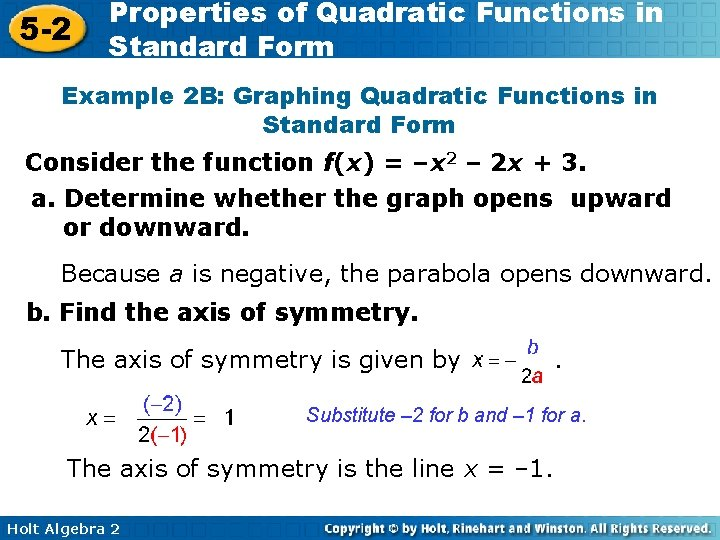5 -2 Properties of Quadratic Functions in Standard Form Example 2 B: Graphing Quadratic Functions in Standard Form Consider the function f(x) = –x 2 – 2 x + 3. a. Determine whether the graph opens upward or downward. Because a is negative, the parabola opens downward. b. Find the axis of symmetry. The axis of symmetry is given by . Substitute – 2 for b and – 1 for a. The axis of symmetry is the line x = – 1. Holt Algebra 25 -2 Properties of Quadratic Functions in Standard Form Example 2 B: Graphing Quadratic Functions in Standard Form Consider the function f(x) = –x 2 – 2 x + 3. c. Find the vertex. The vertex lies on the axis of symmetry, so the x -coordinate is – 1. The y-coordinate is the value of the function at this x-value, or f(– 1) = –(– 1)2 – 2(– 1) + 3 = 4 The vertex is (– 1, 4). d. Find the y-intercept. Because c = 3, the y-intercept is (0, 3). Holt Algebra 2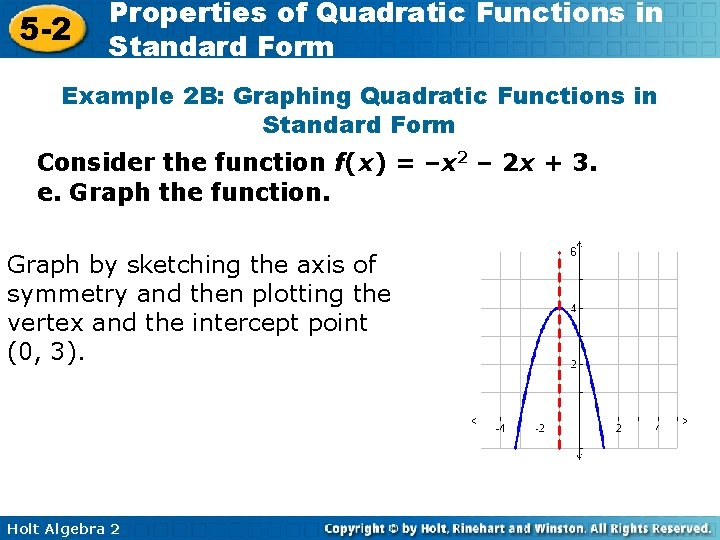5 -2 Properties of Quadratic Functions in Standard Form Example 2 B: Graphing Quadratic Functions in Standard Form Consider the function f(x) = –x 2 – 2 x + 3. e. Graph the function. Graph by sketching the axis of symmetry and then plotting the vertex and the intercept point (0, 3). Holt Algebra 25 -2 Properties of Quadratic Functions in Standard Form Holt Algebra 25 -2 Properties of Quadratic Functions in Standard Form Example 3: Finding Minimum or Maximum Values Find the minimum or maximum value of f(x) = – 3 x 2 + 2 x – 4. Then state the domain and range of the function. Step 1 Determine whether the function has minimum or maximum value. Because a is negative, the graph opens downward and has a maximum value. Step 2 Find the x-value of the vertex. Substitute 2 for b and – 3 for a. Holt Algebra 25 -2 Properties of Quadratic Functions in Standard Form Example 3 Continued Find the minimum or maximum value of f(x) = – 3 x 2 + 2 x – 4. Then state the domain and range of the function. Step 3 Then find the y-value of the vertex, The maximum value is. The domain is all real numbers, R. The range is all real numbers less than or equal to Holt Algebra 25 -2 Properties of Quadratic Functions in Standard Form Example 3 B Find the minimum or maximum value of f(x) = x 2 – 6 x + 3. Then state the domain and range of the function. Step 1 Determine whether the function has minimum or maximum value. Because a is positive, the graph opens upward and has a minimum value. Step 2 Find the x-value of the vertex. Holt Algebra 25 -2 Properties of Quadratic Functions in Standard Form Example 3 B Continued Find the minimum or maximum value of f(x) = x 2 – 6 x + 3. Then state the domain and range of the function. Step 3 Then find the y-value of the vertex, f(3) = (3)2 – 6(3) + 3 = – 6 The minimum value is – 6. The domain is all real numbers, R. The range is all real numbers greater than or equal to – 6, or {y|y ≥ – 6}. Holt Algebra 2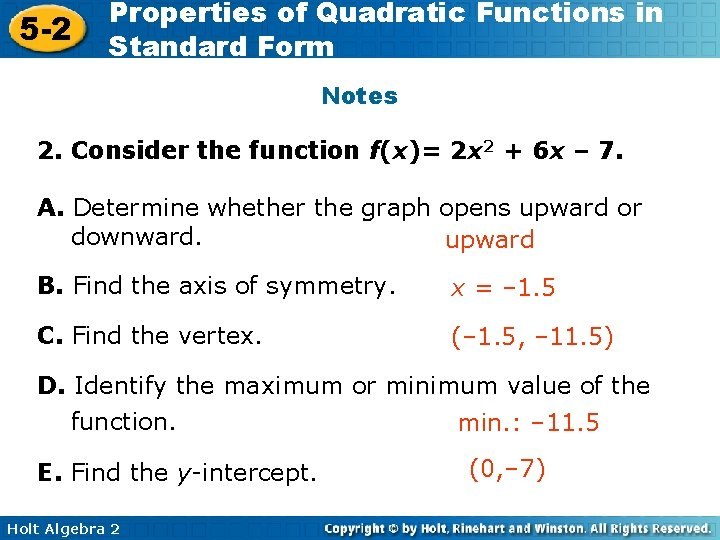5 -2 Properties of Quadratic Functions in Standard Form Notes 2. Consider the function f(x)= 2 x 2 + 6 x – 7. A. Determine whether the graph opens upward or downward. upward B. Find the axis of symmetry. x = – 1. 5 C. Find the vertex. (– 1. 5, – 11. 5) D. Identify the maximum or minimum value of the function. min. : – 11. 5 E. Find the y-intercept. Holt Algebra 2 (0, – 7)5 -2 Properties of Quadratic Functions in Standard Form Notes (continued) Consider the function f(x)= 2 x 2 + 6 x – 7. F. Graph the function. G. Find the domain and range of the function. D: All real numbers; R {y|y ≥ – 11. 5} Holt Algebra 2